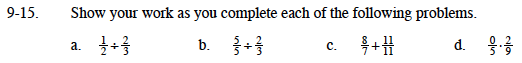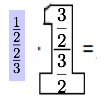### Home > MC1 > Chapter 9 > Lesson 9.1.2 > Problem9-15

9-15.$\frac{\frac{3}{4}}{1}=\frac{3}{4}$

Follow the steps in part (a).

$\frac{11}{11}=1=\frac{7}{7}$

$\frac{0}{5}=0$